Processing ......FreeComputerBooks.com Links to Free Computer, Mathematics, Technical Books all over the World

The R Programming Language
Related Book Categories:
 Statistics and SAS Programming Machine Learning Deep Learning and Neural NetworksProbability and Stochastic Processes Bayesian Thinking Data Analysis and Data Mining, Big Data Data Science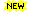Financial Mathematics and Engineering
•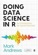Doing Data Science in R: An Introduction for Social Scientists

This approachable introduction to doing data science in R provides step-by-step advice on using the tools and statistical methods to carry out data analysis. It builds knowledge and skills gradually.

•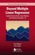Beyond Multiple Linear Regression: Linear & Multilevel Models in R

This book is designed for undergraduate students who have successfully completed a multiple linear regression course, helping them develop an expanded modeling toolkit that includes non-normal responses and correlated structure.

•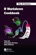R Markdown Cookbook (Yihui Xie, et al)

This book written by the developers of R Markdown is an essential reference that will help users learn and make full use of the software. It will be a popular addition on the desk of many professionals who regularly produce technical documents in the R language.

•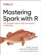Mastering Spark with R: Large-Scale Analysis and Modeling

With this practical book, data scientists and professionals working with large-scale data applications will learn how to use Spark from R to tackle big data and big compute problems.

•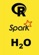Using Spark from R for Performance with Arbitrary Code

This short publication attempts to provide practical insights into using the sparklyr interface to gain the benefits of Apache Spark while still retaining the ability to use R code organized in custom-built functions and packages.

•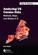Analyzing US Census Data: Methods, Maps, and Models in R

This book is an introduction to geographic data science using R, covers the necessary skills in basic programming, data wrangling and reproducible research to tackle sophisticated but non-spatial data analyses.

•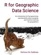R for Geographic Data Science (Stefano De Sabbata)

This book introduces readers to tools in the R programming language for accessing and analyzing Census data from the United States Census Bureau and shows how to carry out demographic analyses in a single computing environment.

•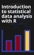Introduction to Statistical Data Analysis with R (Matthias Kohl)

The book offers an introduction to statistical data analysis applying the free statistical software R, probably the most powerful statistical software today. The analyses are performed and discussed using real data.

•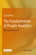The Fundamentals of People Analytics: With Applications in R

Human capital is an organization’s most important asset. Address this need by curating key concepts spanning the entire analytics lifecycle, along with step-by-step instructions for their applications to real-world problems, using open-source software.

•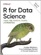R for Data Science: Visualize, Model, Transform, Tidy, Import

This book teaches you how to do data science with R: You'll learn how to get your data into R, get it into the most useful structure, transform it, visualize it and model it, how data science can help you work with the uncertainty and capture the opportunities.

•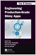Engineering Production-Grade Shiny Apps (Colin Fay, et al)

This book helps people build production-grade Shiny applications, by providing advice, tools, and a methodology to work on web applications with R, with a series of approaches and advice about optimizations for production.

•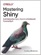Mastering Shiny: Apps, Reports, and Dashboards Powered by R

Master the Shiny web framework - and take your R skills to a whole new level. By letting you move beyond static reports, Shiny helps you create fully interactive web apps for data analyses.

•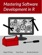Mastering Software Development in R (Roger D. Peng, et al.)

The book covers R software development for building data science tools. As the field of data science evolves, it has become clear that software development skills are essential for producing useful data science results and products.

•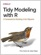Tidy Modeling with R: A Framework for Modeling in the Tidyverse

This book shows data analysts, business analysts, and data scientists how the tidymodels framework offers a consistent, flexible approach for your work. It demonstrate ways to create models by focusing on an R dialect called the Tidyverse.

•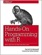Hands-On Programming with R: Functions and Simulations

This book not only teaches you how to program, but also shows you how to get more from R than just visualizing and modeling data. You’ll gain valuable programming skills and support your work as a data scientist at the same time.

•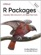R Packages: Organize, Test, Document, and Share Your Code

Turn your R code into packages that others can easily download and use. This practical book shows you how to bundle reusable R functions, sample data, and documentation together by applying author's package development philosophy.

•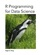R Programming for Data Science (Roger D. Peng)

This book is about the fundamentals of R programming. Get started with the basics of the language, learn how to manipulate datasets, how to write functions, and how to debug and optimize code. You will have a solid foundation on data science toolbox.

•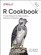R Cookbook: Recipes for Data Analysis, Statistics, and Graphics

This book is full of how-to recipes, each of which solves a specific problem. Each recipe includes a quick introduction to the solution followed by a discussion that aims to unpack the solution and give you some insight into how it works.

•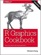R Graphics Cookbook: Practical Recipes for Visualizing Data

This cookbook provides more than 150 recipes to help scientists, engineers, programmers, and data analysts generate high-quality graphs quickly - without having to comb through all the details of R's graphing systems.

•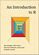An Introduction to R (Alex Douglas, et al.)

The main aim of this book is to help you climb the initial learning curve and provide you with the basic skills and experience (and confidence!) to enable you to further your experience in using R.

•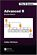Advanced R, Second Edition (Hadley Wickham)

This book helps you understand how R works at a fundamental level. Designed for R programmers who want to deepen their understanding of the language, and programmers experienced in other languages to understand what makes R different and special.

•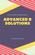Advanced R Solutions (Malte Grosser, et al)

This book offers solutions to the exercises from Advanced R, 2nd Edition by Hadley Wickham. It is work in progress and under active development. The 2nd edition of Advanced R is in print now and we hope to provide most of the answers.

•Efficient R Programming: Practical Guide to Smarter Programming

This book is about increasing the amount of work you can do with R in a given amount of time. It's about both computational and programmer efficiency. This book is for anyone who wants to make their use of R more reproducible, scalable, and faster.

•Cookbook for R: Best R Programming TIPs (Winston Chang)

The goal of this cookbook is to provide solutions to common tasks and problems in analyzing data. Each recipe tackles a specific problem with a solution you can apply to your own project, and includes a discussion of how and why the recipe works.

•Text Mining with R: A Tidy Approach (Julia Silge, et al)

You'll explore text-mining techniques with tidytext, a package that authors developed using the tidy principles behind R packages like ggraph and dplyr. You'll learn how tidytext and other tidy tools in R can make text analysis easier and more effective.

•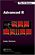Advanced R Programming (Hadley Wickham)

This book presents useful tools and techniques for attacking many types of R programming problems. It not only helps current R users become R programmers but also shows existing programmers what's special about R.

•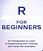R for Beginners (Sasha D. Hafner)

The objective of this book is to introduce participants to the use of R for data manipulation and analysis. It is intented for individuals with little or no prior experience in R. The topics that are covered are the most important for getting started with R.

•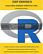Just Enough R: Learn Data Analysis with R in a Day (S. Raman)

Learn R programming for data analysis in a single day. The book aims to teach data analysis using R within a single day to anyone who already knows some programming in any other language.

•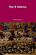The R Inferno (Patrick Burns)

This book is an essential guide to the trouble spots and oddities of R. In spite of the quirks exposed here, R is the best computing environment for most data analysis tasks. If you are using spreadsheets to understand data, switch to R.

•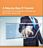A Step-by-Step R Tutorial: Applications and Programming

This book has a two-fold aim: to learn the basics of R and to acquire basic skills for programming efficiently in R. Emphasis is on converting ideas about analysing data into useful R programs.

•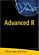Advanced R (Florian Prive)

This book aims at giving a wide understanding of many aspects of R. Combining detailed explanations with real-world examples and exercises, this book will provide you with a solid understanding of both statistics and the depth of R's functionality.

•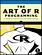The Art of R Programming: A Tour of Statistical Software Design

A guided tour of software development with R, from basic types and data structures to advanced topics like closures, recursion, and anonymous functions. No statistical knowledge is required, and your programming skills can range from hobbyist to pro.

•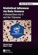Statistical Inference via Data Science: ModernDive, R, Tidyverse

This book provides a pathway for learning about statistical inference using data science tools widely used in industry, academia, and government. It introduces the tidyverse suite of R packages, including the ggplot2 package for data visualization, etc.

•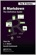R Markdown: The Definitive Guide (Yihui Xie, et al)

With R Markdown, you can easily create reproducible data analysis reports, presentations, dashboards, interactive applications, books, dissertations, websites, and journal articles, while enjoying the simplicity of Markdown and the great power of R and other languages.

•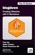blogdown: Creating Websites with R Markdown (Yihui Xie, et al)

Provides a practical guide for creating websites using the blogdown package in R. Use dynamic R Markdown documents to build static websites with automatically rendered output such as graphics, tables, analysis results, and HTML widgets.

•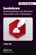bookdown: Authoring Books/Tech Documents with R Markdown

This book presents a much easier way to write books and technical publications than traditional tools such as LaTeX and Microsoft Word. You can make better use of document elements such as figures, tables, equations, theorems, citations, and references.

•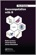Geocomputation with R (Robin Lovelace, et al.)

This book is for people who want to analyze, visualize and model geographic data with open source software. It is based on R, a statistical programming language that has powerful data processing, visualization, and geospatial capabilities.

•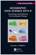Geographic Data Science with R: Visualizing and Analyzing

This book provides a series of tutorials aimed at teaching good practices for using Time Series and geospatial data to address topics related to environmental change. It is based on the R language and aims at data scientists.

•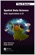Spatial Data Science: With Applications in R (Edzer Pebesma, et al)

Introduces and explains the concepts underlying Spatial Data, gives a detailed explanation of the core spatial software packages for R: sf for simple feature access, and stars for raster and vector data cubes – array data with spatial and temporal dimensions.

•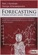Forecasting, Principles and Practice, Using R (R. J. Hyndman)

This textbook provides a comprehensive introduction to forecasting methods and presents enough information about each method for readers to use them sensibly. Examples use R with many data sets taken from the authors' own consulting experience.

•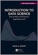Introduction to Data Science: Data Analysis and Algorithms with R

Introduces concepts and skills that can help tackling real-world data analysis challenges. Covers concepts from probability, statistical inference, linear regression, and machine learning. Helps developing skills such as R programming, data wrangling, etc.

•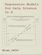Regression Models for Data Science in R (Brian Caffo)

The book gives a rigorous treatment of the elementary concepts of regression models from a practical perspective. The ideal reader for this book will be quantitatively literate and has a basic understanding of statistical concepts and R programming.

•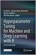Hyperparameter Tuning for Machine and Deep Learning with R

This open access book provides a wealth of hands-on examples that illustrate how hyperparameter tuning can be applied in practice and gives deep insights into the working mechanisms of machine learning (ML) and deep learning (DL) methods.

•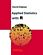Applied Statistics with R (David Dalpiaz)

This book provides an integrated treatment of statistical inference techniques in data science using the R Statistical Software. It provides a much-needed, easy-to-follow introduction to statistics and the R programming language.

•Introduction to Statistical Learning: with Applications in R

It provides an accessible overview of the field of statistical learning, an essential toolset for making sense of the vast and complex data sets that have emerged in fields ranging from biology to finance to marketing to astrophysics in the past twenty years.

•An Introduction to Bayesian Thinking using R

It provides an introduction to Bayesian Inference in decision making without requiring calculus. It may be used on its own as an open-access introduction to Bayesian inference using R Programming for anyone interested in learning about Bayesian statistics.

•Bayes Rules! An Introduction to Applied Bayesian Modeling using R

An engaging, sophisticated, and fun introduction to the field of Bayesian statistics, it brings the power of modern Bayesian thinking, modeling, and computing to a broad audience. Integrates R code, including RStan modeling tools, bayesrules package.

•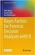Bayes Factors for Forensic Decision Analyses with R

This book provides a self-contained introduction to computational Bayesian statistics using R programming language. With its primary focus on Bayes factors supported by data sets, this book features an operational perspective, practical relevance, and applicability.

•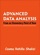Advanced Data Analysis using R (Cosma R. Shalizi)

This is a textbook on data analysis methods, intended for advance undergraduate students who have already taken classes in probability, mathematical statistics, and linear regression. All examples implemented using R.

•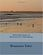Introduction to Statistical Thinking, with R, without Calculus

This is an introduction to statistics, with R, without calculus, for students who are required to learn statistics, students with little background in mathematics and often no motivation to learn more.

•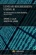Linear Regression Using R: An Introduction to Data Modeling

This book presents one of the fundamental data modeling techniques in an informal tutorial style. Learn how to predict system outputs from measured data using a detailed step-by-step process to develop, train, and test reliable regression models.

•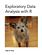Exploratory Data Analysis with R (Roger D. Peng)

This book covers the essential exploratory techniques for summarizing data with R. These techniques are typically applied before formal modeling commences and can help inform the development of more complex statistical models.

•Learning Statistics with R (Daniel Navarro)

This book takes you on a guided tour of software development with R, from basic types and data structures to advanced topics like closures, recursion, and anonymous functions. No statistical knowledge is required.

•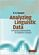Analyzing Linguistic Data: Introduction to Statistics using R

A straightforward introduction to the statistical analysis of language, designed for those with a non-mathematical background. Using the leading statistics programme 'R'. Suitable for all those working with quantitative language data.

•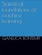Statistical Foundations of Machine Learning using R

This book aims to present the statistical foundations of machine learning intended as the discipline which deals with the automatic design of models from data. All the examples are implemented in the statistical programming language R.

•Statistics with R (Vincent Zoonekynd)

Provides an elementary-level introduction to R, targeting both non-statistician scientists in various fields and students of statistics via code examples with liberal commenting of the code and the output, from the computational as well as the statistical viewpoint.

•Introduction to Probability and Statistics Using R (G. Jay Kerns)

This is a textbook for an undergraduate course in probability and statistics, covers various basic and advanced statistical procedures, from correlation and graph creation to analysis of variance, regression, non-parametric tests, and more.

•R Succinctly (Barton Poulson)

Begin developing your mastery of the powerful R programming language. Become comfortable with the R environment and learn how to find ways for R to fulfill your data needs.

•Using R for Data Analysis and Graphics (J H Maindonald)

This book guides users through the practical, powerful tools that the R system provides. The emphasis is on hands-on analysis, graphical display, and interpretation of data.

•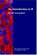An Introduction to R: A Programming Environment for Data Analysis

This tutorial manual provides a comprehensive introduction to R, an open source software package for statistical computing and graphics

•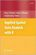Applied Spatial Data Analysis with R (Roger S. Bivand, et al)

It presents R packages, functions, classes and methods for handling spatial data, and showcases more specialised kinds of spatial data analysis, including spatial point pattern analysis, interpolation and geostatistics, areal data analysis and disease mapping.

•A Handbook of Statistical Analyses Using R (Brian S. Everitt, et al)

This book is the perfect guide for newcomers as well as seasoned users of R who want concrete, step-by-step guidance on how to use the software easily and effectively for nearly any statistical analysis.

•Using R for Introductory Statistics (John Verzani)

This book guides students through the basics of R, helping them overcome the sometimes steep learning curve, lays the foundation for further study and development in statistics using R - integrating the study of statistics with a powerful computational tool.

•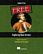Exploring Data Science (Nina Zumel, et al)

This book introduces readers to various areas in data science and explains which methodologies work best for each, with practical examples in R, Python, and other languages.

Book Categories
 :All CategoriesTop Free BooksRecent BooksMiscellaneous BooksComputer EngineeringComputer LanguagesComputer ScienceData Science/DatabasesJava and Java EE (J2EE)Linux and UnixMathematicsMicrosoft and .NETMobile ComputingNetworking and CommunicationsSoftware EngineeringSpecial TopicsWeb Programming
Other Categories